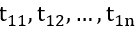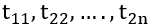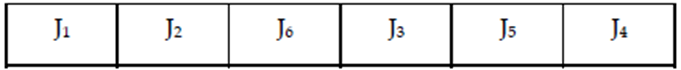Goseeko blog# Sequencing problems: n- jobs, 2 machines

Sequencing: Processing n-jobs through 2 machines Consider n jobs (say 1,2, …n) to be processed on two machines A and B, in the order AB. The processing time isandas represented in Table

Step 1: Check the processing order, i.e., in the order AB or BA. If it is in the order AB, then the first job would be performed on machine A and then on machine B. If the order is of BA, interchange the rows with machine B in row l and machine A in row 2.

Step 2: From the given processing time, select the least processing time available on both machines A and B. If the least processing time exists in row l, place that job at the beginning of the sequence table (i.e., the sequence is from left to right of the sequence table). If the least processing time is on row 2, place the job at the end of the sequence table (i.e., the sequence is from right to left of the sequence table).

Note: If there are two least processing times for machine A and machine B, priority is given for the processing time which has the lowest time of the adjacent machine.

Step 3: Delete the job which has been sequenced, and repeat step 2 until all the jobs are sequenced.

Step 4: Establish a tabular column to determine the total elapsed time and also the idle time for both the machines A and B.

1. Total Elapsed Time: Time when the last job in the sequence has finished on Machine B.

2. Idle Time for Machine A: (Total Elapsed Time) – (Time when the last job has finished on machine B)

3. Idle Time for Machine B: Time at which the first job is finished on machine

Finishes on machine B)

## Example

Six jobs go first over machine I and then over machine II. The order of the completion of jobs has no significance. The table shows the machine times in hours for six jobs and the two machines.

Find the sequence of the jobs that minimizes the total elapsed time to complete the jobs. Also find the idle time for Machine I and Machine II.

Sol:

Establish a sequence table containing six job cells. Find the least time available for both Machine I and Machine II. Job 1 has the least processing time, i.e., 1. Place the sequence from left to right (or the first cell) as shown in Table, since it occurs Machine I.

Deleting job 1, we get the reduced table as shown in Table below

The least time available in the reduced table is 2, which is on Job 4 and Job 5 for Machine II. Now, compare the adjacent time available for Machine I. Here, Job 4 time is less than that for Job 5.

Select Job 4 first and sequence it as shown in Table below:

Now select Job 5 and sequence it as shown in Table below

The reduced table is shown in Table below

Now we have Job 2, Job 3 and Job 6 having the least time which is 3. Compare these times with the adjacent machine time and select the least time. Here we have Job 2 with least adjacent time and hence sequence is as shown below in Table below

Now select Job 3 and sequence it, as shown in Table below

Finally, select Job 6 and sequence it, as shown in Table belowThe optimal sequence thus obtained is

The total elapsed time and idle time for Machine I and Machine II is calculated as shown in Table give below

The total elapsed time is 36 hours

Idle time for Machine I is 4 hours

Idle time for Machine II is 2 hours

nterested in learning about similar topics? Here are a few hand-picked blogs for you!